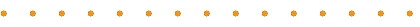## Algorithms and Complexity Group

### Laboratory for Foundations of Computer Science

We are an active research group within the Lab for Foundations of Computer Science, with main research interests in Randomized Algorithms (especially algorithms for sampling and counting), Spectral Graph Theory, Algorithms for Massive Graphs, Computer Algebra, Computational Complexity, Algorithmic Game Theory, Algorithms for Verification, Quantum Complexity and Cryptography, - and with some interest in most aspects of algorithms and complexity. We are always interested in hearing from prospective graduate students (or anyone) with interests in these areas.

During semester-time, we hold an informal "Algorithms reading group" on Wednesdays 4pm-5:30pm.• Mary Cryan
Randomized algorithms (especially for sampling and counting); learning theory; computational biology.
• Heng Guo
Theoretical computer science, especially the complexity of counting problems (for example, computing marginal probabilities and expectations of random variables, the evaluation of partition functions, etc.).
• Kousha Etessami
automated verification, algorithms and computational complexity theory, algorithmic game theory, analysis of probabilistic systems, Markov decision processes, stochastic games, logic, automata theory, model checking, analysis of infinite-state systems, finite model theory.
• Kyriakos Kalorkoti
Computational complexity, computer algebra, decision problems in group theory.
• Richard Mayr
Automated verification, automata and temporal logic, model-checking and semantic equivalence checking, formal verification of real-time and probabilistic systems, infinite-state Markov chains and stochastic games.
• Rik Sarkar
Machine Learning algorithms, Network Analysis, Computational Geometry: topology, and trajectory analysis, Distributed Computing, Data Science.
• He Sun
Algorithmic spectral graph theory, distributed algorithms, data streaming algorithms.

• Jessica Enright
Graph Theory, Networks, and the application of theoretical computer science to controlling the spread of infectious disease.
• Elham Kashefi
Models of quantum computing and their structural relations, exploring new applications, algorithms and cryptographic protocols for quantum information processing devices.

#### Postdoctoral Fellows#### Past Faculty

• Ilias Diakonikolas (now at University of Southern California)
Efficient algorithms for fundamental problems in machine learning.
• Rahul Santhanam (now at Oxford University)
Computational Complexity.
• Amin Coja-Oghlan (now at Frankfurt University)
Random structures and algorithms.
• Mark Jerrum (now at QMUL)
Computational complexity, randomised algorithms, stochastic processes, random structures.